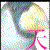### [原创]整数分解随笔（7）

songls1
2019-9-4 21:39
12491

本次文中，未说明的字母，皆为整数，
本次文主要根据同余的一个性质推出一个公式，再根据该公式来讨论整数的分解，如有不对之处，欢迎大家批评指正。

设n=pq，p、q为奇素数，根据同余性质可知：
a≡b(mod n)   =>  a≡b(mod p)
=>  a≡b(mod q)

设n=pq，p、q为奇素数，a^2≡b(mod n),c^2≡d(mod p),其中a=jp+c,j≥1,必有b≡d(mod p),b-a≡d-c(mod p)
证明较简单，把a=jp+c代入a^2≡b(mod n)中，根据同余的性质即可得到，这里不再祥细证明。
该公式说明，对于含有素数p的二次剩余，与p的二次剩余是相同的。

1、对上述公式举例来说明
举例说明：素数23
6^2≡13(mod 23)
即包含素数23的合数n，(j*23+6)^2≡b(mod n)，j≥1，b与23的倍数相差13。
6^2≡13(mod 23) => 6^2≡2*6+1(mod 23)，可得：
(j*23+6)^2≡b(mod n)  => (j*23+6)^2≡b-2*(j*23+6)(mod n)
即b-2*(j*23+6)与23的倍数相差1

29^2≡243(mod 299)，29转换为299两个因子的表达如下：
29=2*13+3,   29=23+6
因为3^2≡9(mod 13),所以243与13的因子234相差为9。
又∵6^2≡13(mod 23),所以243与23的因子230相差13，对于6^2≡13(mod 23)有以下变化：
6^2≡13(mod 23)   =>  6^2≡2*6+1(mod 23)
29^2≡243(mod 299)  => 29^2≡2*29+185
185与23的倍数184相差1
98=4*23+6
98^2≡36(mod 299),36与23相差13
=>98^2≡2*98-160(mod 299)
160与23的倍数161相差1
n=1403=23*61
98^2≡1186(mod 1403),1186与23的倍数1173相差13
=>98^2≡2*98+990(mod 1403)
990与23的倍数989相差1
n=2047=23*89
98^2≡1416(mod 2047),1416与23的倍数1403相差13
=> 98^2≡2*98+1220
1220与23的倍数1219相差1
即素数的二次剩余是不变的。
2、ab≡f(mod n)的作用
对于a^≡b(mod n)，根据b-ja来查找因子，较为困难，如果再增加一个计算值ab≡f(mod n)，那可以根据这几个值的关联关系来查找因子，也许会更快一点。
以下方法是根据相邻三个二次剩余值来查找因子（当然也可以不相邻），仅提供一种思路。
设n=pq，p、q为素数，
(a-1)^2≡d(mod p),a^2≡b(mod p),(a+1)^2≡c(mod p)
对于a^2≡b(mod n),计算a与b的乘积：ab≡f(mod  n)
依据上述几个值关联来求n的因子。
例：n=1411=17*83，以83为例来说明。
30^2≡70(mod 83), 30*70≡25(mod 83)
31^2≡48(mod 83), 31*48≡77(mod 83)
32^2≡28(mod 83), 32*28≡66(mod 83)
依据上述几个值可得如下式子：
28-25=3              ①
77-25*3=2          ②
25*2-48≡2       ③
30-28=2             ④

2*83+30=196^2≡319(mod 1411), 196*319≡440(mod 1411)
2*83+31=197^2≡712(mod 1411), 197*712≡575(mod 1411)
2*83+32=198^2≡1107(mod 1411), 198*1107≡481(mod 1411)

根据①得：1107-440=667, 667与83的倍数664相差3
根据②得：575-440*3=-745, 745与83的倍数747相差2
根据③得：440*2-712=168, 168与83的倍数166相差2
根据④得：196-1107=-911, 911与83的倍数913相差2

83+30,83+31,83+32
3*83+30,3*83+31,3*83+32
4*83+30,4*83+31,4*83+32
.
.
.
皆符合上述的规律，这里不再一一验证，有兴趣的请自行验证(也可以找包含83的合数进行验证)。

还有两个特殊点，一个点为(n-1)/2
对于(n-1)/2的二次剩余有如下的等式：（n=pq，p、q为奇素数）
((n-1)/2)^2≡((p-1)/2)^2(mod p)≡((q-1)/2)^2(mod q)
即(n-1)/2与(p-1)/2和(q-1)/2点相重合。
另一点为k值，n=4k±1,p=4u±1,q=4v±1
k^2≡u^2(mod p)≡v^2(mod q)
即k值也是重合点。

149^2≡75(mod 299)  <=>  6^2≡10(mod 13)
<=> 12^2≡6(mod 23)
75^2≡243(mod 299)  <=>  3^2≡9(mod 13)
<=>  6^2≡13(mod 23)

对于n的二次剩余选点，p值和q值会出现大于(p-1)/2和(q-1)/2的情况，对这种情况只要做n-a，p值与q值的二次剩余就会小于等于(p-1)/2和(q-1)/2，同时也符合对称性的计算，如果把这几个值进行关联，应该可以找到n的因子。
对a^2≡b(mod n)，b-ia中的i值推荐i≤lenth(n)，即i值不大于n的位数。

30^2≡3(mod 299)
4^2≡3(mod 13)
7^2≡3(mod 23)
30=2*13+4=23+7
上述讨论中，c^2≡d(mod p)，即为n=pq中，(jp+c)^2≡b(mod n)，b与p相差d，但上式中，二次剩余都是3，无法求因子，难道0也是因子或者有其它的含义？

对于a^2≡b(mod n),上述给出的是b-ja的算法，但是有时b+ja会快于b-ja
如：n=2047=23*89
128^2≡8(mod 2047)  =>
128+8=136与23的倍数138相差2
但8-128=-120与23的倍数115相差5
所以使用b-ja或使用b+ja哪个更好，目前没有结论，或者两个一起使用，不过计算的工作量将会增加。
同样对ab≡f(mod n)，f与a或b，或者相邻数、二次剩余及乘积，或者n-a及相邻数、二次剩余及乘积，以及对称数的计算，加或减孰优孰劣，或许要具体应用才能知道了。

【公告】看雪招聘大学实习生！看雪20年安全圈的口碑，助你快速成长！Editor2019-9-5 09:26
2
0wendax2019-9-15 22:14
3
0tilamisu2019-9-19 17:06
4
0wendax 容我问一局 a三b(mod n)的三是什么意思ninebell2019-10-6 10:35
5
0songls1 2019-11-16 20:48
6
0songls1 2019-12-15 11:33
7
0

a*r1≡q1(mod p)  其中a*r1>p, a>q1
q1*r2≡q2(mod p)  其中q1*r2>p, q1>q2
q2*r3≡q3(mod p)  其中q2*r3>p, q2>q3
.
.
.
q(n-1)*rn≡1(mod p)  其中q(n-1)*rn>p, q(n-1)>1
以上相乘得：
a*r*q1*r2*...q(n-1)*rn≡q1*q2*...1(mod p)
因假设p为素数，所以上式两边除q1*q2*...得：
a*(r1*r2...rn)≡=1(mod p)
即:a与r1*r2....*rn在p中互逆

例如
求24在83的逆元：
24*4≡13(mod 83)
13*7≡8  (mod 83)
8*11≡5  (mod 83)
5*17≡2  (mod 83)
2*42≡1  (mod 83)
4*7*11*17*42≡45 (mod 83)
即24的逆元为45

求25在83的逆元：
25*4≡17(mod 83)
17*5≡2  (mod 83)
2*24≡1  (mod 83)
4*5*42≡10 (mod 83)
即25的逆元为10

求25在131的逆元：
25*6≡19  (mod 131)
19*7≡2  (mod 131)
2*66≡1  (mod 131)
6*7*66≡21 (mod 131)
即25的逆元为21

求34在131的逆元：
34*4≡5  (mod 131)
5*27≡4  (mod 131)
4*33≡1  (mod 131)
4*27*33≡27 (mod 131)
即34的逆元为27

程序如下：

#include <stdio.h>
main()
{
unsigned long a,b,n;
unsigned long r,q,res;

printf("输入两个数：\n");
scanf("%ld%ld", &a, &n);

b=a;
if( a > n )
{
a=n;
n=b;
}
res=1;
while(1)
{
r=n/a;
r++;
q=r*a-n;
res=(res*r)%n;
if( q == 1 )
{
printf("%ld的逆%ld\n",b,res);
printf("%ld*%ld=%ld(mod %ld)\n",b,res,(res*b)%n,n);
break;
}
if( q == 0 || q == a )
{
printf("%ld存在因子%ld\n",n,a);
break;
}
a=q;
}
}